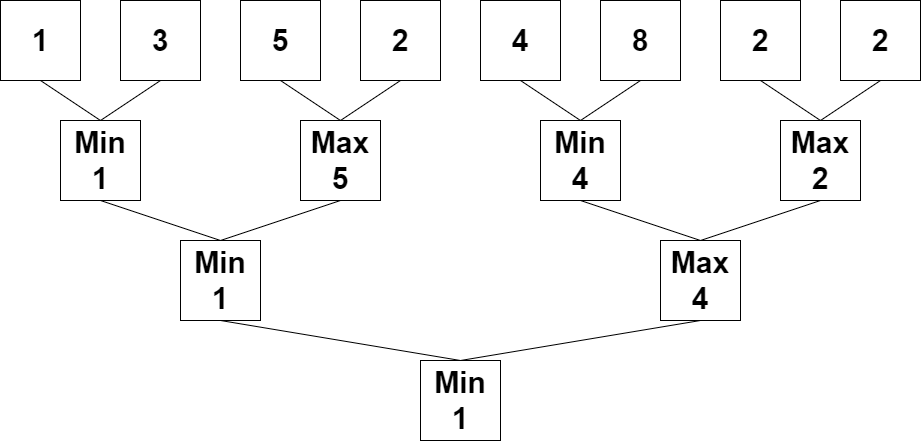2293. Min Max Game

#### QUESTION:

You are given a 0-indexed integer array nums whose length is a power of 2.

Apply the following algorithm on nums:

Let n be the length of nums. If n == 1, end the process. Otherwise, create a new 0-indexed integer array newNums of length n / 2. For every even index i where 0 <= i < n / 2, assign the value of newNums[i] as min(nums[2 * i], nums[2 * i + 1]). For every odd index i where 0 <= i < n / 2, assign the value of newNums[i] as max(nums[2 * i], nums[2 * i + 1]). Replace the array nums with newNums. Repeat the entire process starting from step 1. Return the last number that remains in nums after applying the algorithm.

Example 1:``````Input: nums = [1,3,5,2,4,8,2,2]
Output: 1
Explanation: The following arrays are the results of applying the algorithm repeatedly.
First: nums = [1,5,4,2]
Second: nums = [1,4]
Third: nums = 
1 is the last remaining number, so we return 1.
``````

Example 2:

``````Input: nums = 
Output: 3
Explanation: 3 is already the last remaining number, so we return 3.
``````

Constraints:

``````1 <= nums.length <= 1024
1 <= nums[i] <= 109
nums.length is a power of 2.
``````

#### SOLUTION:

``````class Solution {
func minMaxGame(_ nums: [Int]) -> Int {
var nums = nums
while (nums.count > 1) {
var minFlag = true
var tmpNums:[Int] = []
for index in stride(from: 0, to: nums.count, by: 2) {
if (minFlag) {
tmpNums.append(min(nums[index], nums[index+1]))
} else {
tmpNums.append(max(nums[index], nums[index+1]))
}
minFlag = !minFlag
}
nums = tmpNums
}
return nums
}
}
``````